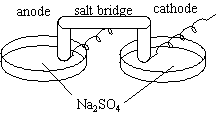# Electrolysis of Water, Version II

$$\newcommand{\vecs}{\overset { \rightharpoonup} {\mathbf{#1}} }$$ $$\newcommand{\vecd}{\overset{-\!-\!\rightharpoonup}{\vphantom{a}\smash {#1}}}$$$$\newcommand{\id}{\mathrm{id}}$$ $$\newcommand{\Span}{\mathrm{span}}$$ $$\newcommand{\kernel}{\mathrm{null}\,}$$ $$\newcommand{\range}{\mathrm{range}\,}$$ $$\newcommand{\RealPart}{\mathrm{Re}}$$ $$\newcommand{\ImaginaryPart}{\mathrm{Im}}$$ $$\newcommand{\Argument}{\mathrm{Arg}}$$ $$\newcommand{\norm}{\| #1 \|}$$ $$\newcommand{\inner}{\langle #1, #2 \rangle}$$ $$\newcommand{\Span}{\mathrm{span}}$$ $$\newcommand{\id}{\mathrm{id}}$$ $$\newcommand{\Span}{\mathrm{span}}$$ $$\newcommand{\kernel}{\mathrm{null}\,}$$ $$\newcommand{\range}{\mathrm{range}\,}$$ $$\newcommand{\RealPart}{\mathrm{Re}}$$ $$\newcommand{\ImaginaryPart}{\mathrm{Im}}$$ $$\newcommand{\Argument}{\mathrm{Arg}}$$ $$\newcommand{\norm}{\| #1 \|}$$ $$\newcommand{\inner}{\langle #1, #2 \rangle}$$ $$\newcommand{\Span}{\mathrm{span}}$$$$\newcommand{\AA}{\unicode[.8,0]{x212B}}$$

## Chemical Concept Demonstrated

• Effects of electrolysis on the acidity of a solution

## Demonstration

 Two crystallizing dishes are filled with Na2SO4; a salt bridge and an attachment to a power supply connect them. Both dishes contain a universal indicator: red, acidic; blue, basic; green, neutral. The current is allowed to run through. The two dishes are combined in a beaker.## Observations

The water turns red at the anode and blue at the cathode. When the dishes are mixed together, the water turns green.

## Explanations (including important chemical equations)

Anode: 2 H2O (l) ---> O2 (g) + 4 H+ (aq) + 4 e - E° = -1.229 V

(solution turns from green to red)

cathode: 2 H2O (l) + 2 e - ---> H2(g) + 2 OH - (aq) E° = -0.828 V
(solution turns from green to blue)

Electrolysis of water produces equivalent amounts of acid and base. Notice that the cathode equation should be multiplied by two in order to conserve the charge in the chemical reaction.

When the dishes are mixed together, the solution is neutral again. Balancing the above equations with respect to the electrons also balances it with respect to the water's ions, so the overall sum of the two dishes is an electrically neutral and pH neutral solution.

Contributors

Electrolysis of Water, Version II is shared under a not declared license and was authored, remixed, and/or curated by LibreTexts.